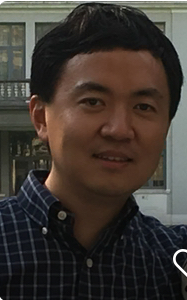Yifei Zhu

I'm an assistant professor at Southern University of Science and Technology (SUSTech) in Shenzhen, China. From 2013 to 2016, I was a visiting assistant professor at Northwestern University. I received my Ph.D. from the University of Minnesota in 2013. My advisor was Tyler Lawson.

My research interests are in algebraic topology and related fields, particularly in its connections to algebraic geometry and number theory via objects such as formal groups, elliptic curves, and modular forms. A central theme in my research is elliptic cohomology and power operations, which combines the classical methodology of cohomological computations in algebraic topology with modern inputs from arithmetic moduli of elliptic curves in number theory, with applications to unstable homotopy. More generally, I study moduli spaces in various aspects, including geometric, arithmetic, computational, combinatorial, categorical. I am also interested in applying topology to physics, such as configuration of line degeneracies in electron energy band structures, and to data science, such as signal processing through persistent homology.

CV

Email: zyf@umn.edu

Conferences: International Workshop on Algebraic Topology

Topology seminar

Papers

Norm coherence for descent of level structures on formal deformations, J. Pure Appl. Algebra 224 (2020), 106382, 35 pp.

Morava E-homology of Bousfield-Kuhn functors on odd-dimensional spheres, Proc. Amer. Math. Soc. 146 (2018), 449--458.

Semistable models for modular curves and power operations for Morava E-theories of height 2, Adv. Math. 354 (2019), 106758, 29 pp.

The Hecke algebra action and the Rezk logarithm on Morava E-theory of height 2, Trans. Amer. Math. Soc. 373 (2020), 3733--3764.

The power operation structure on Morava E-theory of height 2 at the prime 3, Algebr. Geom. Topol. 14 (2014), 953--977.

Presentations

Algebraic topology and arithmetic, Young Mathematician Forum, Beijing International Center for Mathematical Research, Beijing, 2019

Power operations in elliptic cohomology and related arithmetic topics, Special Session on Algebraic and Geometric Topology, AMS-CMS Joint International Meeting, Shanghai, 2018

Toward calculating unstable higher-periodic homotopy types, Electronic Computational Homotopy Theory Seminar, Zoom, 2018

Symmetry encoded by norm maps, International Workshop on Loop Spaces, Supersymmetry and Index Theory, Chern Institute of Mathematics, Tianjin, 2017

Power operation calculations in elliptic cohomology, AMS Special Session on Homotopy Theory, Joint Meetings, Baltimore, 2014

Teaching

MAT8021, Algebraic Topology, Spring 2021

MAT8024, Differentiable Manifolds (in Blackboard), Spring 2020

MA323, Topology

MA327, Differential Geometry, Spring 2019

I've also taught MAT8010, MA301, 102a, 101a at SUSTech and Math 300, 240, 234, 230 and, as a substitute, graduate Algebraic Number Theory at Northwestern.